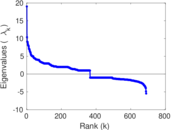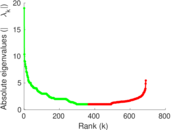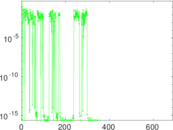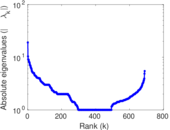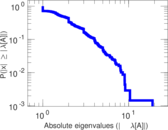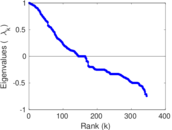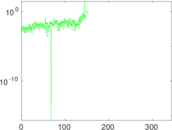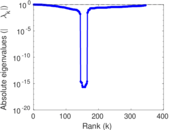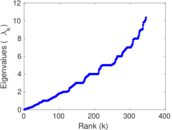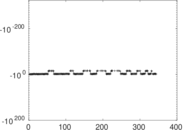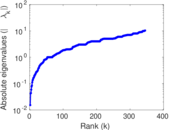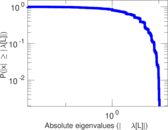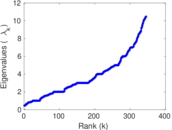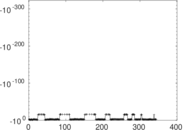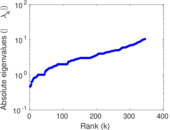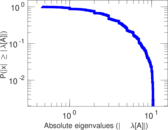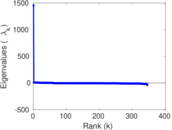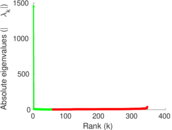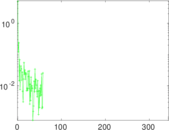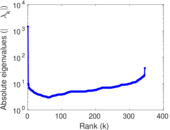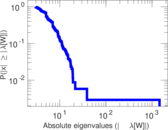Network science

This is a network of co-authorships in the area of network science.

 Code NS Internal name dimacs10-netscience Name Network science Data source https://www.cc.gatech.edu/dimacs10/archive/clustering.shtml AvailabilityDataset is available for download Consistency checkDataset passed all tests Category Co-authorship network Dataset timestamp 2006 Node meaning Author Edge meaning Co-authorship Network format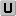Unipartite, undirected Edge typeUnweighted, no multiple edges LoopsDoes not contain loops Join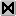Is the join of an underlying network Multiplicity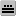Does not have multiple edges, but the underlying data has

Statistics

 Size n = 1,461 Volume m = 2,742 Loop count l = 0 Wedge count s = 16,284 Claw count z = 57,925 Cross count x = 221,122 Triangle count t = 3,764 Square count q = 22,787 4-Tour count T4 = 252,916 Maximum degree dmax = 34 Average degree d = 3.753 59 Fill p = 0.002 570 95 Size of LCC N = 379 Diameter δ = 17 50-Percentile effective diameter δ0.5 = 5.642 87 90-Percentile effective diameter δ0.9 = 9.182 06 Median distance δM = 6 Mean distance δm = 6.285 28 Gini coefficient G = 0.415 264 Balanced inequality ratio P = 0.346 827 Relative edge distribution entropy Her = 0.957 949 Power law exponent γ = 1.970 78 Tail power law exponent γt = 3.611 00 Tail power law exponent with p γ3 = 3.611 00 p-value p = 0.004 000 00 Degree assortativity ρ = +0.461 622 Degree assortativity p-value pρ = 1.231 55 × 10−287 Clustering coefficient c = 0.693 441 Spectral norm α = 19.023 8 Algebraic connectivity a = 0.015 203 8 Spectral separation |λ1[A] / λ2[A]| = 1.833 54 Non-bipartivity bA = 0.712 488 Normalized non-bipartivity bN = 0.255 201 Algebraic non-bipartivity χ = 0.462 123 Spectral bipartite frustration bK = 0.023 953 0 Controllability C = 105 Relative controllability Cr = 0.071 868 6

Plots

Fruchterman–Reingold graph drawing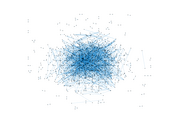Degree distribution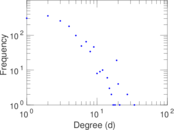Cumulative degree distribution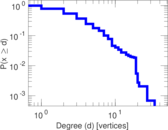Lorenz curve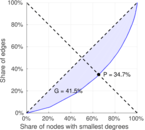Spectral distribution of the adjacency matrix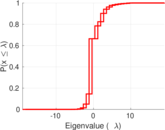Spectral distribution of the normalized adjacency matrix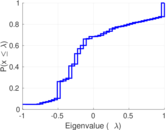Spectral distribution of the Laplacian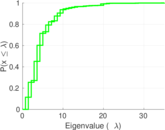Spectral graph drawing based on the adjacency matrixSpectral graph drawing based on the Laplacian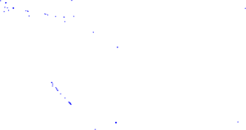Spectral graph drawing based on the normalized adjacency matrix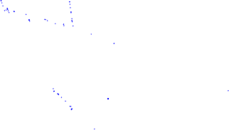Degree assortativity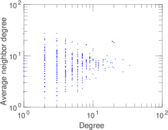Zipf plot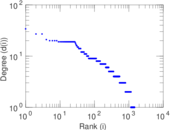Hop distribution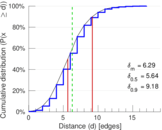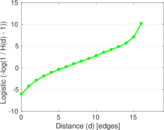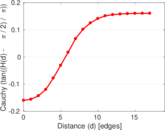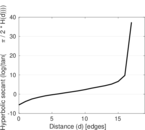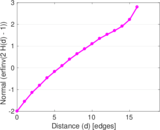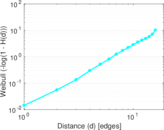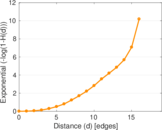Double Laplacian graph drawing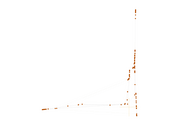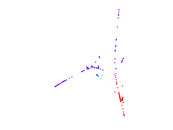Delaunay graph drawing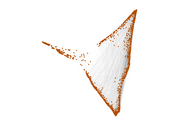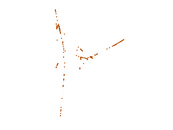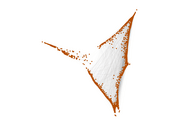Clustering coefficient distribution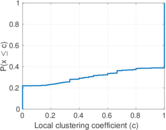Average neighbor degree distribution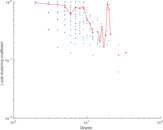SynGraphy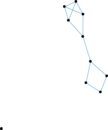Matrix decompositions plots